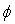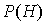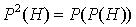NEW TYPES OF TOPOLOGIES

Six new types of topologies have been introduced in the last years (2019-2022), such as: Refined Neutrosophic Topology, Refined Neutrosophic Crisp Topology, NeutroTopology, AntiTopology, SuperHyperTopology, and Neutrosophic SuperHyperTopology.

1)  Refined Neutrosophic Topology

The neutrosophic set has been extended to the Refined Neutrosophic Set (Logic, Probability) , where there are multiple parts of the neutrosophic components, as such T was split into subcomponents T1, T2, ..., Tp, and I into I1, I2, ..., Ir, and F into F1, F2, ...,Fs, with p + r + s = n ≥ 2 and integers t, r, s ≥ 0 and at least one of them is ≥ 2.  Even more: the subcomponents Tj, Ik, and/or Fl can be countable or uncountable infinite subsets of [0, 1].

This definition also includes the Refined Fuzzy Set, when r = s = 0 and p ≥ 2; and the definition of the Refined Intuitionistic Fuzzy Set, when r = 0, and either p ≥ 2 and s ≥ 1, or p ≥ 1 and s ≥ 2.

All other fuzzy extension sets can be refined in a similar way.
The Refined Neutrosophic Topology is a topology defined on a Refined Neutrosophic Set.

{Similarly, the Refined Fuzzy Topology is defined on a Refined Fuzzy Set, while the Refined Intuitionistic Fuzzy Topology is defined on a Refined Intuitionistic Fuzzy Set.

And, as a generalization, on any type of fuzzy extension set [such as" Pythagorean Fuzzy Set, Spherical Fuzzy Set, Fermatean Fuzzy Set, etc.] one can define a corresponding fuzzy extension topology.}

2) Refined Neutrosophic Crisp Topology

The Neutrosophic Crisp Set was defined by Salama and Smarandache in 2014 and 2015.

Let X be a non-empty fixed space. And let D be a Neutrosophic Crisp Set , where D = <A, B, C>, with A, B, C as subsets of X.

Depending on the intersections and unions between these three sets A, B, C one gets several:

Types of Neutrosophic Crisp Sets [2,3]

The object having the form D = is called:

(a) A neutrosophic crisp set of Type 1 (NCS-Type1) if it satisfies: A∩ B = B∩ C = C∩ A =(empty set).

(b) A neutrosophic crisp set of Type 2 (NCS-Type2) if it satisfies: A∩ B = B∩ C = C∩ A =and A∪ B ∪ C = X.

(c) A neutrosophic crisp set of Type 3 (NCS-Type3) if it satisfies: A∩ B ∩ C =and A∪ B ∪ C = X.

Of course, more types of Neutrosophic Crisp Sets may be defined by modifying the intersections and unions of the subsets A, B, and C.

The Refined Neutrosophic Crisp Set was introduced by Smarandache in 2019, by refining/splitting D (and denoting it by RD = Refined D) by refining/splitting its sets A, B, C into sub-subsets as follows:

RD = (A1, ..., Ap; B1, ..., Br; C1, ..., Cs), with p, r, s ≥ 1 be positive integers and at least one of them be ≥ 2, andand many Types of Refined Neutrosophic Crisp

Therefore, the Refined Neutrosophic Crisp Topology is a topology defined on the Refined Neutrosophic Crisp Set.

3) NeutroTopology  is a topology that has at least one topological axiom which is partially true, partially indeterminate, and partially false,

or (T, I, F), where T = True, I = Indeterminacy, F = False, and no topological axiom is totally false, in other words:, where (1, 0, 0) represents the classical Topology, while (0, 0, 1) represents the below AntiTopology.

Therefore, the NeutroTopology is a topology in between the classical Topology and the AntiTopology.

4) AntiTopology  is a topology that has at least one topological axiom that is 100% false (T, I, F) = (0, 0, 1).

The NeutroTopology and AntiTopology are particular cases of NeutroAlgebra and AntiAlgebra  and, in general, they all are particular cases of the NeutroStructure and AntiStructure respectively, since we consider "Structure" in any field of knowledge .

5) SuperHyperTopology  is a topology build on the nth-PowerSet of a given non-empty set H, that excludes the emptyset.

Therefore:is the first powerset of the set H, without the emptyset (Ø);is the second powerset of H (or the powerset of the powerset of H), without the emptysets;

and so on, the n-th powerset of H,, whereis repeated n times ( n ≥ 2 ), and without the emptysets.

6) Neutrosophic SuperHyperTopology  is, similarly, a topology build on the nth-PowerSet of a given non-empty set H, but includes the emptysets [that represent indeterminaciesy] too.

As such, in the above formulas,that excludes the emptyset, is replaced bythat includes the emptyset.is the first powerset of the set H, including the emptyset (Ø);is the second powerset of H (or the powerset of the powerset of H), that includes the emptysets;

and so on, the n-th powerset of H,, where P is repeated n times ( n ≥ 2 ), and includes the emptysets

Conclusion

These six new types of topologies were introduced by Smarandache in 2019-2022, but they have not been much studied and applied yet, except the NeutroTopology and AntiTopology which got some attention from researchers.

References

 F. Smarandache, n-valued Refined Neutrosophic Set and Logic and its Applications in Physics, Progress in Physics, 143-146, Vol. 4, 2013, https://arxiv.org/ftp/arxiv/papers/1407/1407.1041.pdf and http://fs.unm.edu/RefinedNeutrosophicSet.pdf

 A.A. Salama, F. Smarandache, Neutrosophic Crisp Set Theory, Educational Publisher, Columbus, Ohio, USA, 2015; http://fs.unm.edu/NeutrosophicCrispSetTheory.pdf

 Florentin Smarandache, Refined Neutrosophic Crisp Set (RNCS), in the book Nidus Idearum, pp. 114-116, Vol. VII, third edition, 2019, Editions Pons, Brussels, Belgium;

Nidus Idearum book:

Refined Neutrosophic Crisp Set (RNCS) – chapter:

 F. Smarandache, NeutroAlgebra & AntiAlgebra are generalizations of classical Algebras, http://fs.unm.edu/NA/NeutroAlgebra.htm and http://fs.unm.edu/NeutroAlgebra-general.pdf, 2019-2022.

 Florentin Smarandache, Structure, NeutroStructure, and AntiStructure in Science, International Journal of Neutrosophic Science (IJNS), Volume 13, Issue 1, PP: 28-33, 2020;  http://fs.unm.edu/NeutroStructure.pdf

 F. Smarandache, The SuperHyperFunction and the Neutrosophic SuperHyperFunction, Neutrosophic Sets and Systems, Vol. 49, 2022, pp. 594-600, http://fs.unm.edu/NSS/SuperHyperFunction37.pdf

 F. Smarandache, New Types of Topologies and Neutrosophic Topologies, Neutrosophic Systems with Applications, pp. 1-3, Vol. 1, 2023, http://fs.unm.edu/TT/NewTypesTopologies.pdf

 Florentin Smarandache, New Types of Topologies and Neutrosophic Topologies (Improved Version), Neutrosophic Sets and Systems, Vol. 57, 2023, pp. 234-244. DOI: 10.5281/zenodo.8271368MATHEMATICS Algebra Geometries Multispace Neutrosophic Environment Number Theory Statistics Plithogenic Set / Logic /      Probability / Statistics MATHEMATICS Algebra Geometries Multispace Neutrosophic Environment Number Theory Statistics Neutrosophy, a new branch of philosophy PHYSICS Absolute Theory of Relativity Quantum Paradoxes Unmatter Neutrosophic Physics Superluminal and Instantaneous Physics BIOLOGY Neutrosophic Theory of Evolution Syndrome ECONOMICS Poly-Emporium Theory LINGUISTICS Linguistic Paradoxes Linguistic Tautologies PSYCHOLOGY Neutropsychic Personality Illusion Law on Sensations and Stimuli Synonymity Test Complex SOCIOLOGY Social Paradox Sociological Theory LITERATURE pArAdOXisM oUTER-aRT Theatre Literatura Romana Bancuri Science Library Literature Library GlobeTrekker   |   Videos Biography

All articles, books, and other materials from this website are licensed under a
Creative Common Attribute 4,0 International License
,
which permits unrestricted use, distribution, and reproduction in any medium, provided the original work is properly cited.